# GRE Subject Test: Chemistry : Analyzing Data

## Example Questions

← Previous 1

### Example Question #1 : Analytical Chemistry

Robert conducted an experiment in which he investigated how much water a paper towel could absorb. Initially, Robert found that one paper towel can absorb 12.8g of water. Later he found that his scale was not calibrated, so he had to repeat the experiment. After repeating the experiment with a new scale, Robert found that one paper towel can actually absorb 32.9g of water. What is the approximate percent error between the findings of the first and second experiments?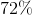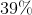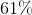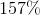Explanation:

The formula for percent error is: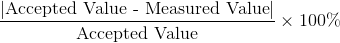In this case, the measured value is 12.8g and the accepted value is 32.9g.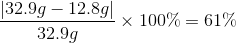### Example Question #1 : Error

A reaction between one mole of sodium and one mole of chloride should yield 42 grams of sodium chloride. In your experiment, the actual yield is 32.73 grams. Calculate the percent error of your experiment.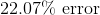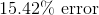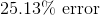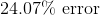Explanation:

To find percent error we need to use the following equation: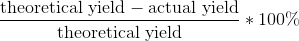Plug in 42 for the theoretical yield and 32.73 for the actual yield and solve accordingly.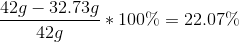### Example Question #1 : Calculating Error

In the following reaction, eight moles of sodium hydroxide is broken down into four moles of sodium oxide and four moles of water. What is the percent error if your experiment yields 195 grams of sodium oxide?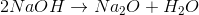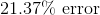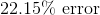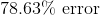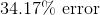Explanation:

To find the percent error we need to use the following equation:But in order to do this, we first have to convert moles of sodium oxide into grams: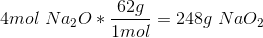This gives us a theoretical yield of 248g, which we plug in with our 195g actual yield.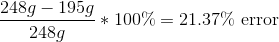### Example Question #1 : Analyzing Data

If a given sample of silver and flourine ideally combine to form 0.6mol of AgF, what is the percent error if the actual yield is 43 grams?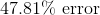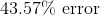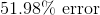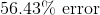Explanation:

Our first step to complete this problem is to convert moles AgF into grams: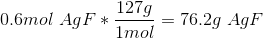This gives us a theoretical yield of 76.2 grams. We can find the percent error by plugging in 76.2g for our theoretical yield and 43g for the actual yield in the following equation: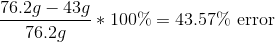### Example Question #1 : Understanding Accuracy And Precision

Michael buys several bags of balloons. On the package, it says that each bag has 100 balloons. He opens the bags and only one of them has 100 balloons inside; the other bags either have too many or too few.

How would you describe the bag of balloons with 100 balloons inside?

Neither accurate nor precise

Accurate, but not precise

Accurate, but the precision cannot be determined

Both accurate and precise

Precise, but not accurate

Accurate, but not precise

Explanation:

This bag is accurate because it provided the correct number of balloons, however, the process is not precise as the results were clearly not repeatable.

Accuracy deals with how close the measurement got to the accepted measurement. Precision deals with how consistent the measurement is. The bag with 100 balloons inside matched the claim made on the bag, meaning it was accurate. It was not precise because the other measurements show that the number of balloons is variable.

### Example Question #1 : Understanding Accuracy And Precision

Michael's scale measures the mass of objects as consistently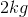less than their actual mass. How would you describe the scale?

Accuracy and precision are synonyms

It is neither accurate nor precise

It is both accurate and precise

It is accurate, but not precise

It is precise, but not accurate

It is precise, but not accurate

Explanation:

Precision measures is how consistently a device records the same answer. In this case, Michael's scale is ALWAYSshort. Even though it displays the wrong value, it is consistent. That means it is precise. Measuring a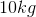object will always display a mass of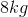; the results are easily reproduced.

Accuracy is how well a device measures something against its accepted value. In this case, Michael's scale is not accurate because it is always off by.

### Example Question #1 : Analyzing Data

An brand of fruit snacks claims that each bag of fruit snacks has a mass of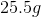. After weighing three bags, Wally observes the masses to be,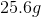, and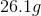.

How can Wally describe the accuracy and precision of the first bag he measured?

There are insufficient data points to draw a conclusion

Precise, but not accurate

Neither accurate nor precise

Accurate and precise

Accurate, but not precise

Accurate, but not precise

Explanation:

The claim for the mass of the first bag is accurate; the brand says there should bein each bag and there wasin the first bag.

The claim on the first bag is not precise, as the results are not replicated universally throughout the experiment. The masses of the bags fluctuate, with the average of all three bags equal to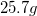.

### Example Question #2 : Analyzing Data

A bowman is shooting arrows at a target. Which of the following demonstrates high accuracy but low precision?

The bowman consistently hits to the left of the target

The bowman consistently hits the bullseye

The bowman consistently misses the target and hits a tree in the same spot

The bowman consistently hits around the target but never hits the bullseye

The bowman consistently hits to the right of the bullseye

The bowman consistently hits around the target but never hits the bullseye

Explanation:

Accuracy is measured as the degree of closeness to the actual measurement. In our case, accurate shots will hit the bullseye. Precision is measured as the degree of closeness of one measurement to the next. In our case, precise shots will be clustered together.

To get high accuracy but low precision, measurements must center around the target value but be variable. The bowman's arrows will not be clustered (low precision), but will be accurately distributed around the bullseye. If all the shots were averaged, the bullseye would be at the center.

### Example Question #2 : Analytical Chemistry

Which of these is an example of high precision?

A student tries to throw a pencil into the garbage can and makes it in

A student correctly calculates the mass of an object to be 54kg

An archer hits the bulls-eye

A student correctly calculates the acceleration due to gravity to be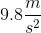An archer hits the same spot on the target three times in a row

An archer hits the same spot on the target three times in a row

Explanation:

Precision is a measure of reproducibility. If multiple trials produce the same result each time with minimal deviation, then the experiment has high precision. This is true even if the results are not true to the theoretical predictions; an experiment can have high precision with low accuracy.

In contrast, accuracy is the measure of difference between a calculated value and the true value of a measurement. High accuracy demands that the experimental result be equal to the theoretical result.

An archer hitting a bulls-eye is an example of high accuracy, while an archer hitting the same spot on the bulls-eye three times would be an example of high precision.

### Example Question #3 : Understanding Accuracy And Precision

Which of these is an example of high accuracy?

A student throws three pencils into the trash can, but misses and hits the window each time

A student calculates the acceleration due to gravity to be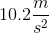using three different methods

An archer hits the same spot on the target three times in a row

An archer hits the bulls-eye

A student repeatedly calculates the mass of an object to be 49 grams, when the true mass is 52 grams

An archer hits the bulls-eye

Explanation:

Accuracy is the measure of difference between a calculated value and the true value of a measurement. High accuracy demands that the experimental result be equal to the theoretical result.

In contrast, precision is a measure of reproducibility. If multiple trials produce the same result each time with minimal deviation, then the experiment has high precision. This is true even if the results are not true to the theoretical predictions; an experiment can have high precision with low accuracy.

An archer hitting a bulls-eye is an example of high accuracy, while an archer hitting the same spot on the bulls-eye three times would be an example of high precision.

← Previous 1

### All GRE Subject Test: Chemistry Resources